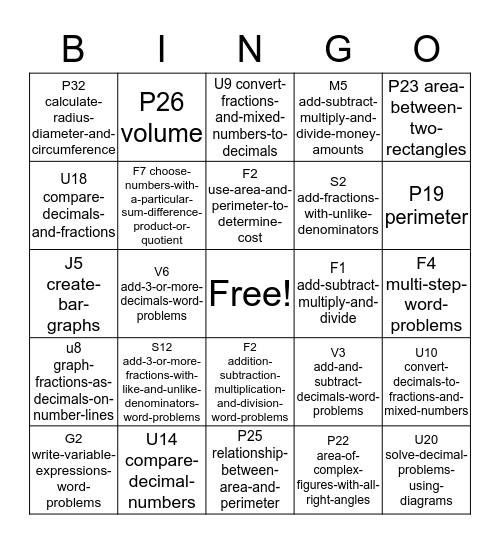# IXL Bingo 4This bingo card has a free space and 24 words: S12 add-3-or-more-fractions-with-like-and-unlike-denominators-word-problems, U14 compare-decimal-numbers, F2 use-area-and-perimeter-to-determine-cost, G2 write-variable-expressions-word-problems, P23 area-between-two-rectangles, P32 calculate-radius-diameter-and-circumference, U18 compare-decimals-and-fractions, P26 volume, F1 add-subtract-multiply-and-divide, F2 addition-subtraction-multiplication-and-division-word-problems, S2 add-fractions-with-unlike-denominators, J5 create-bar-graphs, V3 add-and-subtract-decimals-word-problems, U10 convert-decimals-to-fractions-and-mixed-numbers, F7 choose-numbers-with-a-particular-sum-difference-product-or-quotient, P22 area-of-complex-figures-with-all-right-angles, F4 multi-step-word-problems, U9 convert-fractions-and-mixed-numbers-to-decimals, P19 perimeter, V6 add-3-or-more-decimals-word-problems, U20 solve-decimal-problems-using-diagrams, u8 graph-fractions-as-decimals-on-number-lines, M5 add-subtract-multiply-and-divide-money-amounts and P25 relationship-between-area-and-perimeter.

## Play Online

Share this URL with your players:For more control of your online game, create a clone of this card first.

## Probabilities

With players vying for a you'll have to call about __ items before someone wins. There's a __% chance that a lucky player would win after calling __ items.

Tip: If you want your game to last longer (on average), add more unique words/images to it.